# Team:KU Leuven/Project/Ecological/Modelling

iGem

### Secret garden

Congratulations! You've found our secret garden! Follow the instructions below and win a great prize at the World jamboree!

• A video shows that two of our team members are having great fun at our favourite company. Do you know the name of the second member that appears in the video?
• For one of our models we had to do very extensive computations. To prevent our own computers from overheating and to keep the temperature in our iGEM room at a normal level, we used a supercomputer. Which centre maintains this supercomputer? (Dutch abbreviation)
• We organised a symposium with a debate, some seminars and 2 iGEM project presentations. An iGEM team came all the way from the Netherlands to present their project. What is the name of their city?

Now put all of these in this URL:https://2013.igem.org/Team:KU_Leuven/(firstname)(abbreviation)(city), (loose the brackets and put everything in lowercase) and follow the very last instruction to get your special jamboree prize!We present to you our ecological model. Ultimately our project aims to reduce crop loss because of aphid infestation. Given the time span of the competition, it is impossible to conduct field experiments. Therefore we attempt to predict the effect of our pheromones on the surroundings through a series of modelling steps. Eventually, we might be able to calculate the optimal spacing of the stickers with BanAphids to be maximally effective.

The first step is to calculate the concentration of the pheromones released in the environment. When a colony of BanAphids is placed in a field, the produced substances (pheromones, ...) will be transported in the air by diffusion and convection. Diffusion is almost always present, whereas the convection term depends on the presence of a source. In our case the wind is responsible for convection, so we searched an appropriate term for wind convection. To establish a realistic model, certain parameters are needed. Therefore, approximate diffusion coefficients and air velocity were searched. Production of the pheromones by the colony was coupled with another modelling approach.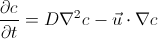The formula above is commonly known as the convection-diffusion equation, which is a partial differential equation in c (the concentration of the pheromones in our case). D is the diffusion coefficient and u the velocity of the solvent (which is the air).

### Diffusion coefficients

Because we found no measured diffusion coefficients of the pheromones in literature, estimations were made with a calculator based on methods described in (Lyman, Reehl & Rosenblatt, 1982). Using the average supplied by the calculator, the results are 4.62 x 10-6 m2/s for E-beta-farnesene and 6.33 x 10-6 m2/s for methyl salicylate. The conditions supplied were a pressure of 1 atm and a temperature of 15 °C.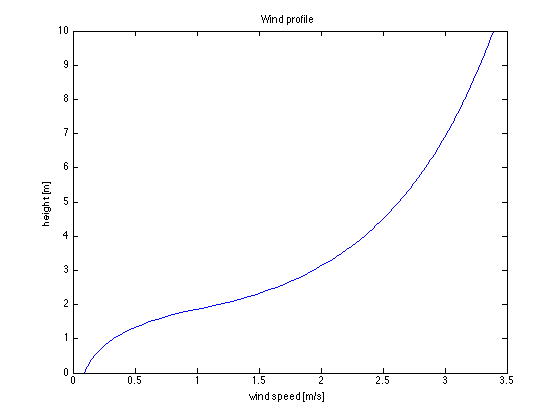Figure 1 ǀ Wind profile for a crop height of 2 m and a wind speed of 3.39 m/s at a height of 10 m.

### Wind speed

Because of friction and obstacles on the earth’s surface, wind speed varies with altitude. Generally, the velocity increases with increasing altitude. A logarithmic wind profile is appropriate for the part above the crops (Goudriaan, 1977, p. 96). The formula for this profile is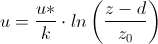with u representing the velocity. Here d accounts for an upward shift above a vegetative cover. The relation d=0.63 x zc is suggested, where zc is the height of the crops. The length z0 is called the roughness length and is often supposed to be about one tenth of zc.

For the part inside the canopy, the profile is exponential.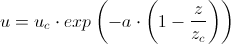As can be noticed, the wind speed decreases exponentially with extinction factor a when approaching ground level and thus going deeper into the canopy. uc is the speed at height zc and can easily be calculated by the formula of the logarithmic wind profile.

Evidently, the wind direction changes in time. Most regions however tend to have a dominant wind (“Prevailing winds,” Wikipedia, 2013), at least during a certain time period. This is the reason our model only incorporates convection in one direction, which greatly simplifies calculation. Furthermore, average wind speeds are measured and published monthly by the Royal Meteorological Institute (KMI) in Belgium (“Maandelijkse normalen - KMI,” 2013). These are measured at 10 m above the ground, making it possible to calculate u*/k used in the log law. Now, all parameters for convection are known when the crop height is given. The wind profile was entered in the software using a piecewise function and self-defined parameters, making it easy to change wind speed and crop height. An example of the profile is plotted in Figure 1.

### Boundary conditions

In order to solve our model, boundary conditions need to be specified. We'll represent the environment as a square box (5x5x5m) with 6 faces. Within this environment box, we assume a bacterium box (5x5x1cm), suspended in "mid air", with its own 6 (smaller) faces.

#### Inflow and outflow

For the environment box, the faces perpendicular to the wind direction were specified as an inflow (with a concentration of 0 mol/m3), respectively an outflow.

#### No flux

Within the environment box, the face representing the ground will have no flux through it, because we assume that the diffusion coefficient of our pheromones is much larger in soil than in air. Within the bacterium box, all faces but one (pheromone outlet) are given zero flux as well.

#### Open boundaries

The remaining 3 faces of the environment box remain "open" since the pheromone flux through these faces is unknown at this point.

#### Specified flux

The upper face of the bacterium container is the only place where pheromones are released in the air. Under the assumption that the pheromone production and vaporisation is at steady state, we can set the flux at this surface equal to the production rate of the entire colony.

Once we know the production per cell (by the procedure in MeS Modelling) it is straightforward to calculate the output of the whole colony by using an average cell density in the appropriate growth phase. This output represented as an amount per time can then be converted to a flux through the contact surface with the air, which can be entered in the simulation program.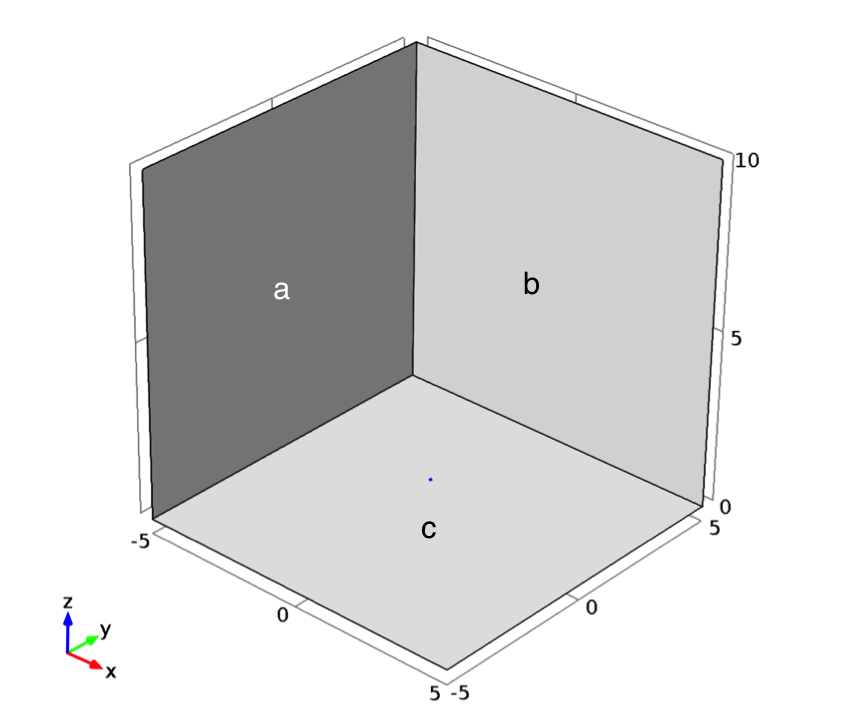Figure 2 ǀ Geometry for the model: the blue dot is the container with BanAphids, a is an influx plane, b an open boundary and c a face with no flux.

### Results

Although we lacked real values for the production rates of the pheromones, we ran the model with a "ballparked" flux of 1 mmol/(m2s) to get an idea of the shape of the solution. We used a simple raised cosine function to capture the oscillating behaviour of the oscillator with a period of 1 hour. As can be seen in the animated figure below, convection by wind is the dominant effect on dispersion of the pheromones. Therefore the solution follows the oscillations quite well and lag or local accumulation of pheromones is not a problem. Note that the solution has negative values at some points, which is physically impossible of course. This is solely due to numerical errors and can be avoided by the use of a finer mesh (at a large computational cost).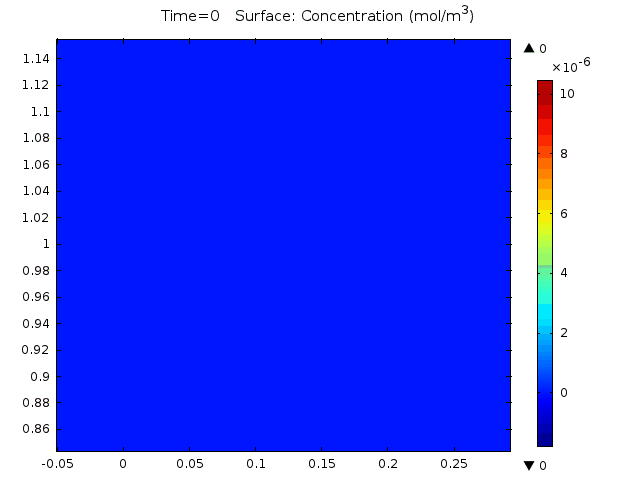Figure 3 ǀ Vertical slice of the geometry showing the concentration of methyl salicylate. Time is in seconds.

One of the missing parameters in our model was the sensitivity of aphids for E-beta-farnesene. No values for the air concentration threshold could be found in literature, so we decided to use our model to calculate this. From the work of (Nault, Edwards & Styer, 1973) we found that the effect of E-beta-farnesene released by aphids can reach up to 3 cm far. Using the real-time emission analysis of (Schwartzberg et al., 2008) we fitted a decreasing exponential function through these points. The result of this is (0.34+0.7273*exp(-0.109/60*t))/2 ng/s for one aphid. To use this as a flux boundary condition in our model, some conversions have to be done. First, we'll assume that the pheromone is released from a cornicle represented by a sphere with a radius of 0.1 mm. When combining this with the molar mass of EBF, the result is 0.00012233*(0.17+0.36365*exp(-0.00181667*t))/π mol/(m2.s).
When looking at a distance of 3 cm from the "cornicle" we find an average EBF air concentration of 3.36*10-6 mol/m3, which can be taken as the threshold value for aphid EBF perception. These values can be used towards calculating the optimal distance between stickers, placed in individual plants. Please check out the practical uses below for further details.

### Software

At first, we used Mathworks Matlab with the Partial Differential Equations Toolbox, but this software was limited to 2D geometries and more stringent boundary conditions. Later on, we noticed that the Department of Chemical Engineering of our university provided COMSOL Multiphysics. This program was very suitable for our purposes, as it provides a model for “Transport of Diluted Species”. Our complete model can be downloaded here.Apart from the shape of the pheromone cloud which will arise, more interesting conclusions can be drawn from these results.

Above, we found the threshold concentration for which the aphids are sensitive. Using this and the production rate per sticker, we can calculate how far apart the stickers with BanAphids can be. The opposite is also possible: if we would like to have a specific distance between the stickers, it is possible to find the appropriate colony size in one sticker.

We can even go further and integrate data about the behaviour and reproduction of the insects to create a population model. This would allow to fully measure the effect on the environment and the crops.

Goudriaan, J. (1977). Crop micrometeorology : a simulation study. Wageningen University, Wageningen.
Lyman, W. J., Reehl, W. F., & Rosenblatt, D. H. (1982). Handbook of chemical property estimation methods: environmental behavior of organic compounds. McGraw-Hill.
Maandelijkse normalen - KMI. (n.d.). Meteo. Retrieved August 22, 2013, from http://www.meteo.be/meteo/view/nl/65239-Home.html
Prevailing winds. (2013, August 21). In Wikipedia, the free encyclopedia. Retrieved from http://en.wikipedia.org/w/index.php?title=Prevailing_winds&oldid=569524163
Nault LR, Edwards LJ, Styer WE (1973) Aphid alarm pheromones: secretion and reception. Environ Entomol 2: 101–105.
Schwartzberg et al. (2008), “Real-Time Analysis of Alarm Pheromone Emission by the Pea Aphid (Acyrthosiphon Pisum) Under Predation,” Journal of Chemical Ecology 34, no. 1: 76–81